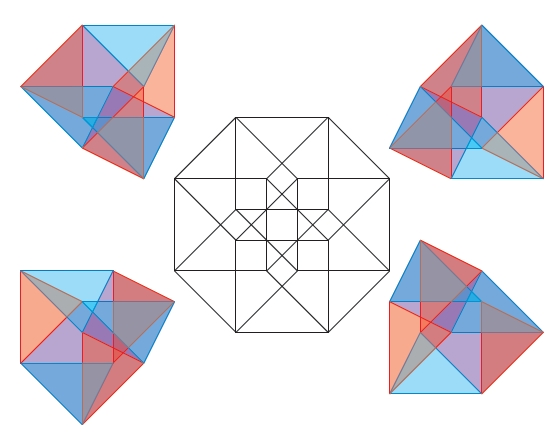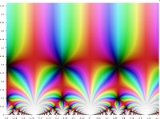## February 15, 2011

### Heron’s Formula

#### Posted by John Baezguest post by J. Scott Carter

An undergraduate student, David Mullens, and I recently posted a paper that presents a 4-dimensional proof of Heron’s formula. (Caution: it’s about 2.5 megabytes in size.)

You may recall from your high school geometry class that Heron’s formula tells how to compute the area of a triangle given its side lengths. The form that I want to present the formula is as follows:

$16 A \times A = (a+b+c)(a+b-c)(a-b+c)(-a+b+c)$

where $A$ indicates the area of the triangle, and the side lengths are $a, b$, and $c$. By convention we choose variables so that $a$ is no greater than $b$ which is no greater than $c$. Traditionally, Heron’s formula is stated in terms of the semi-perimeter, but our purpose is to demonstrate that two hyper-volumes (4-dimensional analogues of volume) are actually scissors congruent.

Our motivations came from various conversations in various places. So I will relate the personal details before the technical details.

Abhijit Champanerkar and I indicated in this paper a higher dimensional interpretation of the fact that the integral of an $n$th power (over the unit interval) is the $(n+1)$-dimensional volume of a pyramidal structure whose base is the $n$-cube. We did not know of the paper of Nils Barth, Computing Cavalieri’s Quadrature Formula By a Symmetry of the n-Cube, American Mathematical Monthly, 111 (2004), no. 9, 811-813, who had discovered essentially the same thing, so we have let our manuscript sit on the arXiv.

The proof that Barth gives and that we give is a nice dinner-time or tea-time story that can be related to the mathematician who is higher-dimensionally inclined. During one such conversation in Hanoi, Dylan Thurston asked us if we knew of a $4$-dimensional proof that the sum of the cubes of consecutive integers is the square of the corresponding triangular number. From the point of view of using Bernoulli numbers to sum $k$th powers, the context of Dylan’s question is quite natural. Dylan reported the proof which he, Dave Bayer, and Walter Neumann had discovered at a dinner conversation. The proof involved splitting the cube into two prisms, taking the cone on each and reassembling them into the hyper-solid that is a triangle times a triangle.

The conversation with Dylan stimulated me to use a $2$-dimensional projection of a right isosceles triangle times the same as a model for some artistic sketches that were made in homage to the artist Tony Robbin. These appear here . At a conference on art and math in Tampa, Florida, I showed some of these works as part my slides and mentioned the mathematics of the preceding two paragraphs to John H. Conway over dinner. At that point, Conway mentioned that he sought a 4-dimensional proof of Heron’s formula.

Mullens, who is a mathematics and statistics major at the University of South Alabama, had taken to posting short problems on a hallway blackboard in a “Good Will Hunting” sort of a way. The problems were various jewels from a variety of math problem solving books that we keep available in the department. A few of these had to do with applications of Heron’s formula, and so I gave Mullens the Heron’s formula problem to work upon.

The proof in our paper relies upon a scissors congruence proof of the Pythagorean Theorem. Any such proof is sufficient, but we explicitly use the one in which a square with edge length $z$ is decomposed into four right triangles (with legs of length $x$ and $y$) and a central square of edge length $y-x$, as indicated here for example.

It is fairly easy to see that the distributive law is a scissors congruence, and we spent some time in the paper to discuss the scissors congruence properties when negative edge lengths are involved.

Our major lemma is that if polygons $P$ and $Q$ are respectively scissors congruent to $P'$ and $Q'$, then the hyper-solids $P \times Q$ and $P' \times Q'$ are scissors congruent. This result follows, roughly, from the fact that cartesian products distribute over unions. More explicitly, we examine dihedral angles between facets of the hyper-solids. Within the figures, we develop in detail the scissors congruence for the product of two proofs of the Pythagorean theorem.

Finally, we examine the high school algebra proofs in terms of the scissors congruences outlined above. The expression $16 A^2$, where $A$ is the area of the triangle, represents the volume of four hyper-parallelograms (parallelogram times parallelogram), each of which is decomposed into four copies of a triangle times triangle. The expression $(a+b+c)(a+b-c)(a-b+c)(-a+b+c)$ is the hyper-volume of a hyper-rectangle. It is decomposed into $81$ pieces via the distributive law. All but nine terms in this expression cancel. Some of those nine terms will further decomposed using the proof of the Pythagorean theorem.

Posted at February 15, 2011 4:30 AM UTC

TrackBack URL for this Entry:   https://golem.ph.utexas.edu/cgi-bin/MT-3.0/dxy-tb.fcgi/2358

### Re: Heron’s Formula

Readers who enjoy higher-dimensional proofs by dissection should go back and look at our blog entry on Categorifying Nichomachus of Gerasa’s Equation.

Here’s a pretty picture from Scott Carter’s new paper showing how the usual dissection of a square into triangles gives a dissection of a hypercube into four shapes, each the product of two triangles.It’s about the simplest picture in the paper!

Posted by: John Baez on February 15, 2011 5:19 AM | Permalink | Reply to this

### Re: Heron’s Formula

Very nice! It reminded me ever so slightly of this three-dimensional proof of a two-dimensional fact about circles. (Not sure there’s really a lot in common apart from the use of higher dimensions though.)

Posted by: Tom Leinster on February 15, 2011 10:50 AM | Permalink | Reply to this

### Re: Heron’s Formula

Are you perhaps thinking about Desargues’ theorem?

Posted by: David Mullens on February 25, 2011 7:50 AM | Permalink | Reply to this

### Re: Heron’s Formula

Nevermind…the first time I clicked the link “this” it didn’t bring me to the same place it brought me with the second click…so scratch that

Posted by: David Mullens on February 25, 2011 7:54 AM | Permalink | Reply to this

### Re: Heron’s Formula

Scott,

Posted by: jim stasheff on February 15, 2011 2:03 PM | Permalink | Reply to this

### Re: Heron’s Formula

Jim,

I certainly consider the Heron’s formula paper to be math, but some of the other stuff on my web site might be considered art.

Posted by: Scott Carter on February 15, 2011 3:02 PM | Permalink | Reply to this

### Re: Heron’s Formula

I agree, but I’m most impressed by the interesection

Posted by: jim stasheff on February 16, 2011 1:21 PM | Permalink | Reply to this

### Re: Heron’s Formula

This is about triangle. Are the methods of any use in the generalizations of Heron’s formula like the famous Brahmagupta’s formula for quadrilateral. There are even some formulas beyond that where also the computational algebra is very useful. See

• Dragutin Svrtan, Darko Veljan, Vladimir Volenec, Geometry of pentagons: from Gauss to Robbins, arxiv:math.MG/0403503
Posted by: Zoran Skoda on February 15, 2011 8:01 PM | Permalink | Reply to this

### Re: Heron’s Formula

David is working on Brahmagupta’s formula while taking his classes. My hope is that our techniques will follow simply. Thanks for the link to the pentagon’s papers.

Posted by: Scott Carter on February 15, 2011 11:01 PM | Permalink | Reply to this

### Re: Heron’s Formula

Heron’s formula, and its higher dimensional analogues, can be derived from the Cayley-Menger determinant.

An amazing fact I learned recently, while reading an article by David Hestenes, is that the matrix in the Cayley-Menger determinant can be seen as a Gramian matrix of 5 vectors in 5 dimensional anti-DeSitter space:R is the circumradius, with (x00, y00,z00) the circumcenter.
In other words:,I’m trying to figure out what this means!

I’m using this in a theory of electric networks.

Gerard

Posted by: Gerard Westendorp on February 23, 2011 10:47 PM | Permalink | Reply to this

### Re: Heron’s Formula

[one of the formula went wrong]

Heron’s formula, and its higher dimensional analogues can be derived from the Cayley-Menger determinant.

An amazing fact I learned recently, while reading an article by David Hestenes, is that the matrix in the Cayley-Menger determinant can be seen as a Gramian matrix of 5 vectors in 5 dimensional Anti de Sitter space:R is ther circumradius, with (x00, y00,z00) the circumcenter.
In other words:,I’m trying to figure out what this means!

I’m using this in a theory of electric networks.

Gerard

Posted by: Gerard Westendorp on February 23, 2011 10:52 PM | Permalink | Reply to this

### Re: Heron’s Formula

A really good structure determination program is the XPLOR-NIH. It also allows to perform efficient molecular dynamics and minimizations!

Best regards,
Ronald

Posted by: Ronald Suttersberg on March 6, 2011 12:23 PM | Permalink | Reply to this

### Re: Heron’s Formula

For a nice link between Heron’s formula and elliptic curves, see this webpage. The moral is:

The space of triangles which share the same area and perimeter forms an elliptic curve!

The group law actually helps one to write down explicit pairs of such triangles. Very nifty if one is trying to explain elliptic curves to high school students…

Posted by: Bruce Bartlett on March 9, 2011 3:21 PM | Permalink | Reply to this

### Re: Heron’s Formula

Interesting.
This related to another thing I’ve been trying to figure out, and which has also been subject on this forum. It may also lead to understanding of the connection between modular forms and elliptic functions. (thinking of course secretly about Fermat’s last theorem here)

I’ve been making tilings of Riemann surfaces on one of my web pages. A missing piece is how to put complex functions on the tiles, such that they satisfy a modular equation, such as
X3Y + Z3X + Y3Z = 0

These functions would look something like the j-invariant:The j-invariant can be thought of as being composed of triangular tiles, each of which has all the complex numbers mapped on it exactly once. The j-invariant is an automorphic function of the modular group, related to modular forms, so finding automorphic functions of tilings is related to modular forms. But from your suggestion it appears they are also quite directly related to elliptic curves.

The simplest modular curve is X + Y + Z = 0. But this equation is precisely the requirement for a triangle, formed by 3 complex numbers (X,Y,Z) interpreted as 2 dimensional vectors. What I need is a nice way to assign a value of X, Y, and Z to all points of a triangle, such that all complex numbers appear just once. In other words, map the complex plane onto a triangle in 3 different ways. I could do it with a Schwarz–Christoffel mapping, but I’d like to have a nice geometric interpretation to go with it. Maybe the space of triangles will help to find it.

Gerard

Posted by: Gerard Westendorp on March 15, 2011 12:48 AM | Permalink | Reply to this

Post a New Comment